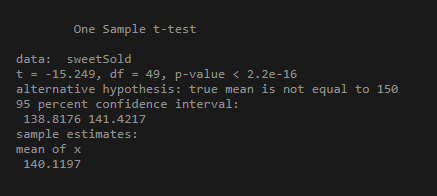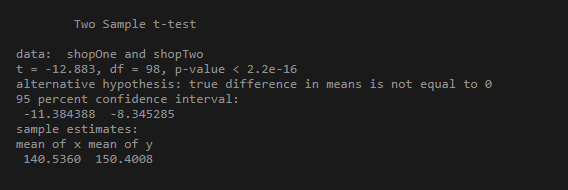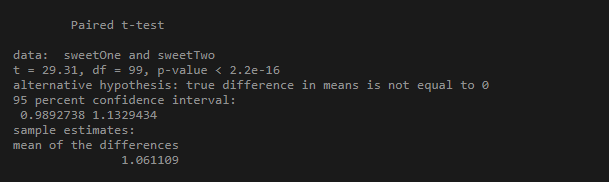# T-Test Approach in R Programming

We will be trying to understand T-Test in R Programming with the help of an example. Suppose a businessman with two sweet shops in a town wants to check if the average number of sweets sold in a day in both the stores is the same or not.
So, the businessman takes the average number of sweets sold to 15 random people in the respective shops. He found out that the first shop sold 30 sweets on average whereas the second shop sold 40. So, from the owner’s point of view, the second shop was doing better business than the former. But the thing to notice is that the data-set is based on a mere number of random people and they cannot represent all the customers. This is where T-testing comes into play it helps us to understand that the difference between the two means is real or simply by chance.

Mathematically, what t-test does is, it takes a sample from both the sets and establishes the problem assuming a null hypothesis that the two means are the same.

#### Classification of T-tests

• One Sample T-test
• Two sample T-test
• Paired sample T-test

#### One Sample T-test

The One-Sample T-Test is used to test the statistical difference between a sample mean and a known or assumed/hypothesized value of the mean in the population.
So, for performing a one-sample t-test in R, we would use the syntax `t.test(y, mu = 0) ` where x is the name of the variable of interest and mu is set equal to the mean specified by the null hypothesis.
For Example:

 `set``.seed(``0``) ` `sweetSold <``-` `c(rnorm(``50``, mean ``=` `140``, sd ``=` `5``)) ` `t.test(sweetSold, mu ``=` `150``) ``# Ho: mu = 150 `

Output:#### Two sample T-test

It is used to help us to understand that the difference between the two means is real or simply by chance.
The general form of the test is `t.test(y1, y2, paired=FALSE)`. By default, R assumes that the variances of y1 and y2 are unequal, thus defaulting to Welch’s test. To toggle this, we use the flag var.equal=TRUE.

For Example:

 `set``.seed(``0``) ` ` `  `shopOne <``-` `rnorm(``50``, mean ``=` `140``, sd ``=` `4.5``) ` `shopTwo <``-` `rnorm(``50``, mean ``=` `150``, sd ``=` `4``) ` ` `  `t.test(shopOne, shopTwo, var.equal ``=` `TRUE) `

Output:#### Paired Sample T-test

This is a statistical procedure that is used to determine whether the mean difference between two sets of observations is zero. In a paired sample t-test, each subject is measured two times, resulting in pairs of observations.

The test is run using the syntax `t.test(y1, y2, paired=TRUE)`

For Example:

 `set``.seed(``2820``) ` ` `  `sweetOne <``-` `c(rnorm(``100``, mean ``=` `14``, sd ``=` `0.3``)) ` `sweetTwo <``-` `c(rnorm(``100``, mean ``=` `13``, sd ``=` `0.2``)) ` ` `  `t.test(sweetOne, sweetTwo, paired ``=` `TRUE) `

Output:My Personal Notes arrow_drop_upCheck out this Author's contributed articles.

If you like GeeksforGeeks and would like to contribute, you can also write an article using contribute.geeksforgeeks.org or mail your article to contribute@geeksforgeeks.org. See your article appearing on the GeeksforGeeks main page and help other Geeks.

Please Improve this article if you find anything incorrect by clicking on the "Improve Article" button below.

Article Tags :

2

Please write to us at contribute@geeksforgeeks.org to report any issue with the above content.